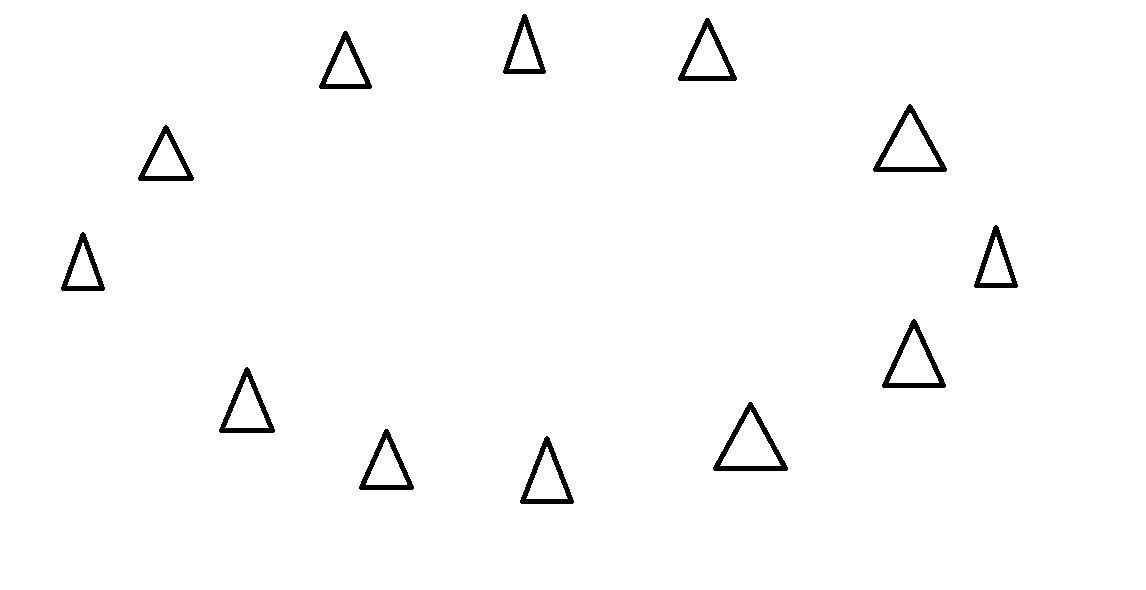## Multivariate Statistics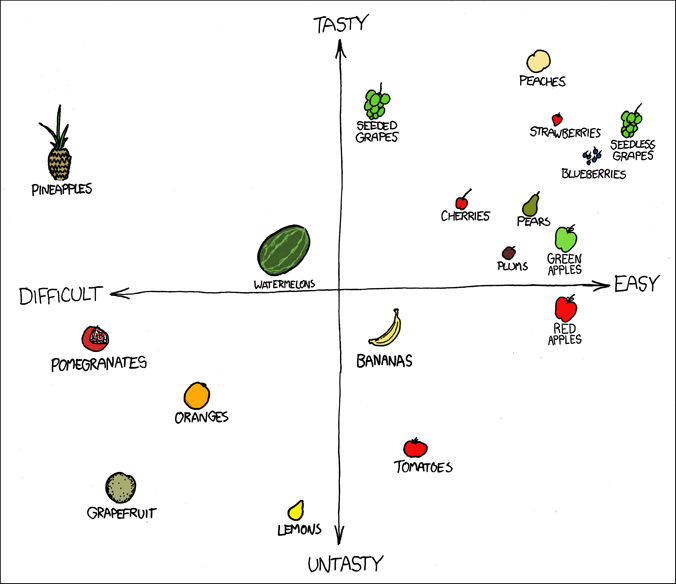## A brief note on presentationsEvery time someone says "I know you can't read the axis labels", a pygmy marmoset is murdered.

## Multivariate Data

Often we collect many different variables

We want to answer questions like:

• how are the variables related?
• are their differences in the means and variances of the variables?
• can we look at a composite of some of these variables to simplify our data?

These questions are the domain of multivariate statistics

## Matrix Algebra

The nuts and bolts of multivariate statistics

You need to know the basics (at least the terminology) in order to understand multivariate stats.

## A matrix

$A = \left[\begin{array}{cccc} a_{11} & a_{12} & .. & a_{1n} \\ a_{21} & a_{22} & .. & a_{2n} \\ .. & .. & .. & ..\\ a_{m1} & a_{m2} & .. & a_{mn} \end{array}\right]$

• Consists of $$m$$ rows and $$n$$ columns
• if $$m=n$$ then it is a square matrix
• each row is a row vector
• each column is a column vector
• a single number is called a scalar
• matrices are represented by capital letters

## Matrix Transposition

Matrices can be transposed by swapping the rows and columns

$A = \left[\begin{array}{cc} a & b\\ c & d \\ e & f \end{array}\right]$

becomes

$A' = \left[\begin{array}{ccc} a & c & e\\ b & d & f \end{array}\right]$

## Types of Matrices

Zero matrix $0 = \left[\begin{array}{ccc} 0 & 0 & 0\\ 0 & 0 & 0 \\ 0 & 0 & 0\end{array}\right]$

Diagonal matrix $D = \left[\begin{array}{ccc} d_1 & 0 & 0\\ 0 & d_2 & 0 \\ 0 & 0 & d_3\end{array}\right]$

A Symmetrical matrix is a square matrix whose transpose is identical to the original.

## Types of Matrices

Diagonal matrix $D = \left[\begin{array}{ccc} d_1 & 0 & 0\\ 0 & d_2 & 0 \\ 0 & 0 & d_3\end{array}\right]$

Identity matrix $I = \left[\begin{array}{ccc} 1 & 0 & 0\\ 0 & 1 & 0 \\ 0 & 0 & 1\end{array}\right]$

## Operations on Matrices

Equality - Two matrices are equal only if they have same size, and all elements are equal.

Trace - The sum of the diagonal terms.

Addition, subtraction, multiplication and division all have their matrix counterparts.

## Eigenvalues and Eigenvectors

Consider a system of linear equations, where $$\lambda$$ is a scalar:

$a_{11}x_1 + a_{12}x_2 + ... + a_{1n}x_n = \lambda x_1 \\ a_{21}x_1 + a_{22}x_2 + ... + a_{2n}x_n = \lambda x_2\\ ... \\ a_{n1}x_1 + a_{n2}x_2 + ... + a_{nn}x_n = \lambda x_n$

We can write this in matrix form.

$Ax = \lambda x$ or $(A - \lambda I)x = 0$

## Eigenvalues and Eigenvectors

These equations only hold true for some values of $$\lambda$$, which are called the eigenvalues.

There are up to $$n$$ eigenvalues.

These equations can be solved for a given eigenvalue (e.g., the $$ith$$), and the resulting set of values is called the $$ith$$ eigenvector.

The sum of the eigenvalues of matrix $$A$$ is equal to the trace of matrix $$A$$.

## Covariance Matrices

Recall the sample covariance for two variables, calculated from the sum of cross-products

$\ s_{XY} = \frac{\sum\limits_{i=1}^n(X_i - \bar{X})(Y_i - \bar{Y})}{(n-1)}$

## Covariance Matrices

With a multivariate sample comprising $$p$$ variables, we can define a sample covariance matrix:

$S = \left[\begin{array}{ccc} s_{11} & s_{12} & .. & s_{1p}\\ s_{21}& s_{22} & .. & s_{2p} \\ .. & .. & .. & .. \\ s_{p1} & s_{p2} & .. & s_{pp} \end{array}\right]$

What do the diagonal elements represent?

## Covariance Matrices

x <- rnorm(100)
y <- x * 0.3 + rnorm(100, sd=0.4)
z <- rnorm(100)
myMatrix <- cbind(x,y,z)
var(myMatrix)
##            x           y           z
## x 0.82922999  0.25469090  0.03235001
## y 0.25469090  0.23848375 -0.03049321
## z 0.03235001 -0.03049321  0.96998485

## Correlation Matrices

Recall the correlation coeffiecient for two variables, which is a scaled version of the covariance.

$correlation\ coeffiecient = \frac{cov\ XY}{(sd\ X \times sd\ Y)}$

or more formally:

$r = \frac{s_{xy}}{(s_{X} \times s_{Y})}$

## Correlation Matrices

We can then compute the correlation matrix for a multivariate sample of $$p$$ variables.

$R = \left[\begin{array}{cccc} 1 & r_{12} & .. & r_{1p}\\ r_{21}& 1 & .. & r_{2p} \\ .. & .. & .. & .. \\ r_{p1} & r_{p2} & .. & 1 \end{array}\right]$

Why are the diagonal elements all 1?

## Correlation Matrices

head(myMatrix, 4)
##               x          y           z
## [1,]  0.1232968 -0.5625834  0.32971941
## [2,] -0.4176242 -0.1091754 -1.16895205
## [3,]  0.4861737 -0.1354634 -0.03478491
## [4,] -0.4668539 -0.2972959 -1.80087286
cor(myMatrix)
##            x          y           z
## x 1.00000000  0.5727255  0.03607069
## y 0.57272551  1.0000000 -0.06340030
## z 0.03607069 -0.0634003  1.00000000

## Multivariate Distance Metrics

With a single variable (e.g. femur length) it is easy to conceptualize how far apart two observations are.

• Femur A = 25cm
• Femur B = 30cm
• How far apart are these individuals in terms of femoral length?

As we add more measurements, it becomes less obvious to tell how "far apart" two specimens are.

If the Humerus of indivual A is 15cm and the Humerus of indiviaual B is 22cm, then how far apart are individuals A and B?

We need a multivariate distance metric.

## Euclidian Distance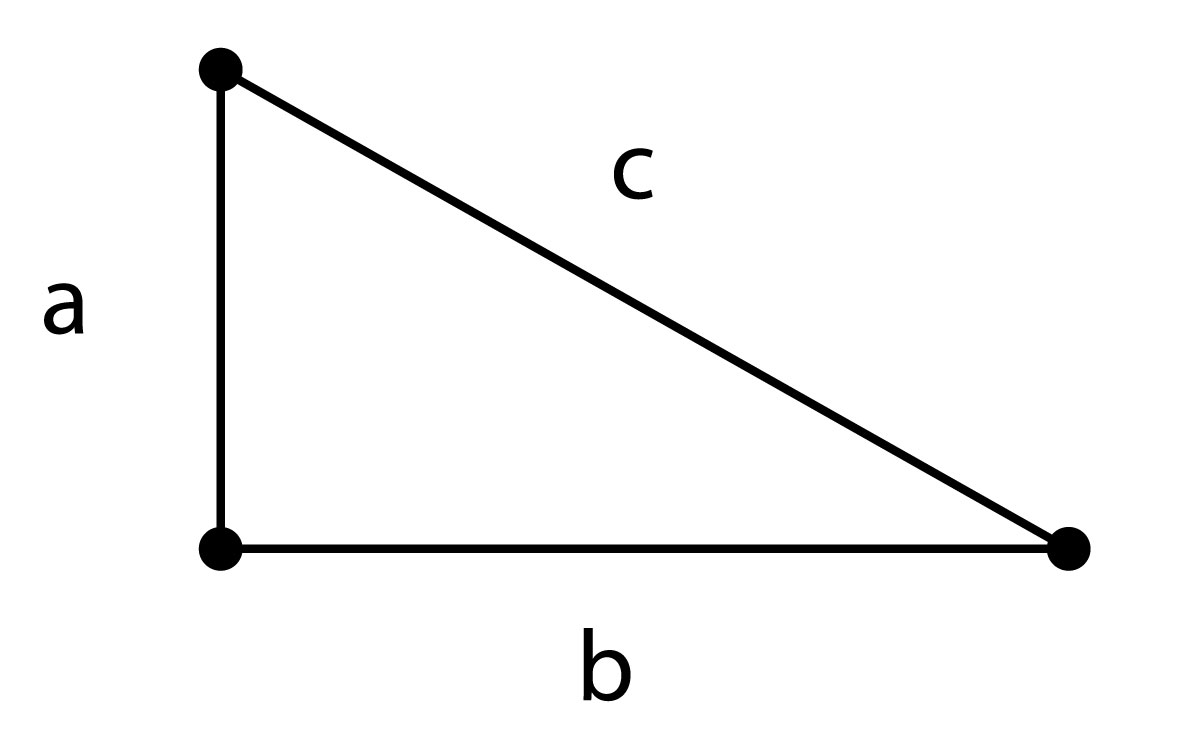$c = \sqrt{a^2 + b^2 }$

## Euclidian Distance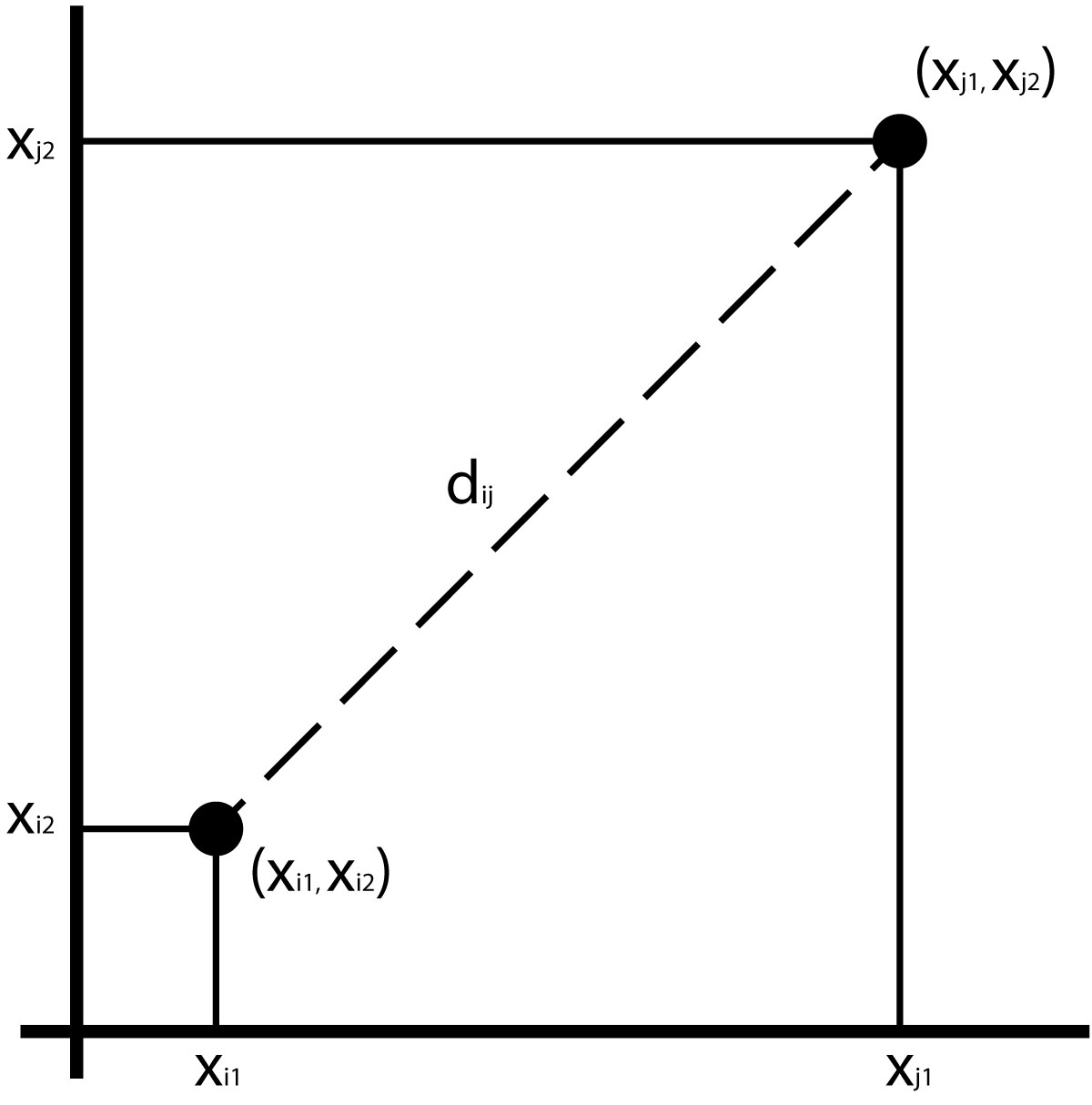Assuming 2 variables, we can compute the distance as the hypotenuse of a triangle:

$d_{ij} =\sqrt{(x_{i1} - x_{j1})^2 + (x_{i2} - x_{j2})^2}$

## Euclidian Distance

We can compute a Euclidian distance for any number of variables:

$d_{ij} = \sum\limits_{k=1}^p \sqrt{ (x_{ik})^2 - (x_{jk})^2 }$

## Euclidian Distance

Euclidian distances can easily be swamped by large scale measurements.

To avoid this, you can calculate a z score by subtracting the mean of the measurement and dividing by the standard deviation.

The dist() function in R calculates Euclidian distances by default.

## Mahalanobis Distance

Calculates the distance of an observation from its multivariate sample, taking into account the covariance of the variables.

$D^2_{ij} = \sum\limits_{r=1}^p \sum\limits_{s=1}^p (x_{r} - \mu_{r})\ v^{rs} (x_{s} - \mu_{s})$

where $$v^{rs}$$ is the covariance between variables $$r$$ and $$s$$

## Mahalanobis Distance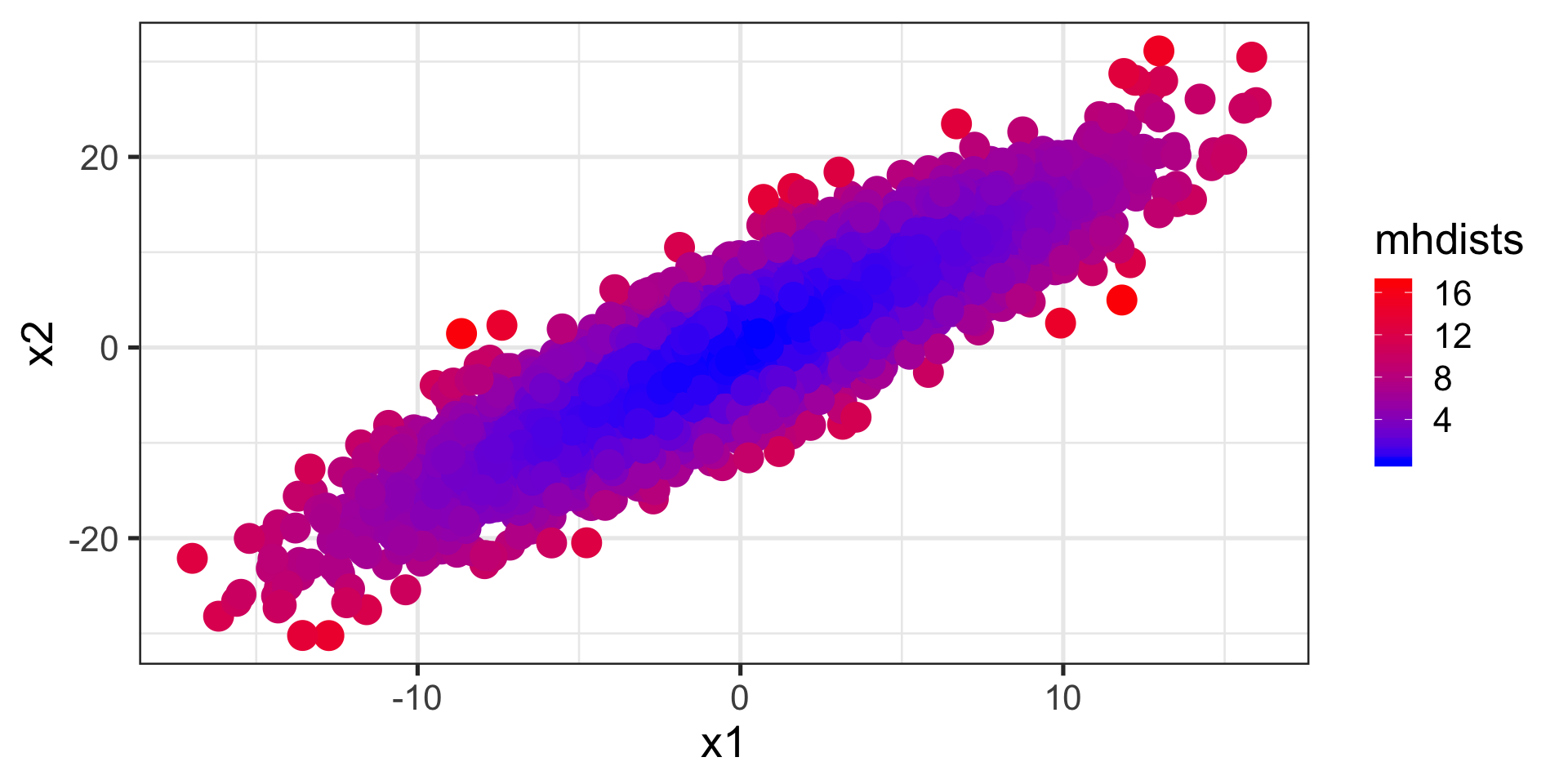## Mahalanobis Distance

means <- c(mean(x1), mean(x2))
myMatrix <- cbind(x1, x2)
VCV <- var(myMatrix)
mhdists <- mahalanobis(myMatrix, means, VCV)
mhdists[1:4]
##  2.1335285 0.1871506 2.1965283 5.6212356

## Distance Matrices

Several multivariate techiques derive directly from matrices of distances.

## Calculate a Euclidian Distance Matrix

var1 <- rnorm(5); var2 <- rnorm(5)
myMatrix <- cbind(var1,var2); head(myMatrix, 3)
##            var1       var2
## [1,] -1.8168975 -0.4935965
## [2,]  0.6271668 -2.1222441
## [3,]  0.5180921 -0.1335666
dist(myMatrix)
##           1         2         3         4
## 2 2.9369957
## 3 2.3625829 1.9916665
## 4 1.9589314 1.7630234 0.4782398
## 5 3.3253874 2.3607936 0.9649143 1.4136512

## Cluster Analysis

assume each individual is in a group of 1

join individuals within a given distance into a group

continue joining groups until there is a single group

visualize with a tree (dendrogram)

## Principal Components Analysis

Goal is to "summarize" variation in multivariate data

Reducing dimensionality of dataset is called ordination and many multivariate techniques fall in to this category

Reduce the number of dimensions needed to describe most of the variance

## PCA - Intuitively